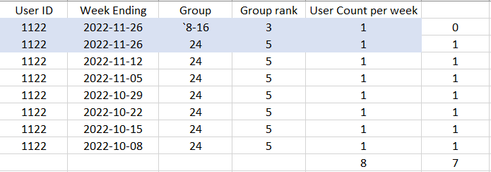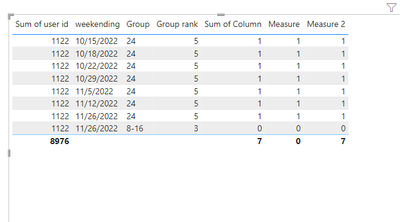cancel
Showing results for
Did you mean:Frequent Visitor

## How to calculate higher value for same dates on user level

Hi all,

I need to solve problem below presented: I am trying to calculate count of rows by unique users but problem is when user appears two or more times in same week. In example below, in #2 groups, 8-16 and 24 (highlighted in light-blue)

The output of this problem, in order to present number correctly should be like in unnammed column, meaning that if one user appears more that once in same week, I should count only the highiest group. In this case, for week 2022-11-26, only to consider group 24 as 1 and 8-16 as 0, so my easy countdistinct formula will show 7 instead of 8.1 ACCEPTED SOLUTIONCommunity Support

Hi @NebojsaZ88 ,

Please refer to my pbix file to see if it helps you.

Create measures.

``````Measure =
IF (
MAX ( [Group rank] )
= CALCULATE (
MAX ( 'Table'[Group rank] ),
FILTER ( ALL ( 'Table' ), [weekending] = SELECTEDVALUE ( 'Table'[weekending] ) )
),
1,
0
)
``````
``````Measure2 =
VAR _b =
SUMMARIZE ( 'Table', 'Table'[weekending], "aaa", [Measure] )
RETURN
IF ( HASONEVALUE ( 'Table'[weekending] ), [Measure], SUMX ( _b, [aaa] ) )
``````

Or create a column.

``````Column =
IF (
[Group rank]
= CALCULATE (
MAX ( 'Table'[Group rank] ),
FILTER ( 'Table', [weekending] = EARLIER ( 'Table'[weekending] ) )
),
1,
0
)
``````If it does not help, please provide more details with your desired output and pbix file without privacy information (or some sample data) .

Best Regards
Community Support Team _ Polly

If this post helps, then please consider Accept it as the solution to help the other members find it more quickly.

2 REPLIES 2Community Support

Hi @NebojsaZ88 ,

Please refer to my pbix file to see if it helps you.

Create measures.

``````Measure =
IF (
MAX ( [Group rank] )
= CALCULATE (
MAX ( 'Table'[Group rank] ),
FILTER ( ALL ( 'Table' ), [weekending] = SELECTEDVALUE ( 'Table'[weekending] ) )
),
1,
0
)
``````
``````Measure2 =
VAR _b =
SUMMARIZE ( 'Table', 'Table'[weekending], "aaa", [Measure] )
RETURN
IF ( HASONEVALUE ( 'Table'[weekending] ), [Measure], SUMX ( _b, [aaa] ) )
``````

Or create a column.

``````Column =
IF (
[Group rank]
= CALCULATE (
MAX ( 'Table'[Group rank] ),
FILTER ( 'Table', [weekending] = EARLIER ( 'Table'[weekending] ) )
),
1,
0
)
``````If it does not help, please provide more details with your desired output and pbix file without privacy information (or some sample data) .

Best Regards
Community Support Team _ Polly

If this post helps, then please consider Accept it as the solution to help the other members find it more quickly.Frequent Visitor

Hi @v-rongtiep-msft ,

With small changes (ALLEXCEPT instead of ALL) I managed to get result + I added into FILTER User ID as my table contains 1000+ unique users (I just show here example for one). Overall, good guidance to what I wanted, so thank you. 👌

``````Measure =
IF (
MAX ('Table'[Group rank]) =
CALCULATE (
MAX ('Table'[Group rank]),
FILTER (ALLEXCEPT('Table','Table'[Week Ending]), 'Table'[User ID] = SELECTEDVALUE('Table'[User ID]))),1,0)``````

But, with second measure, I have result i wanted for matrix viz, but when I want to show as chart (live printscreen below) and in Y-axis put measure 2, there is no values, why?Announcements#### Exclusive opportunity for Women!

Join us for a free, hands-on Microsoft workshop led by women trainers for women where you will learn how to build a Dashboard in a Day!#### Power Platform Conference-Power BI and Fabric Sessions

Join us Oct 1 - 6 in Las Vegas for the Microsoft Power Platform Conference.Top Solution Authors
Top Kudoed Authors
Users online (1,599)